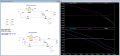# In loop compensation OPA377

#### sunney

Joined Sep 3, 2015
23
Hi,

Below are two voltage buffer circuits with and without compensation using OPA377.
AC analysis is done using break-the-loop method where
Vout/Vfb = AOL (open loop gain)
Vout = AOL x beta = Close loop gain
1/Vfb = feedback factor

https://www.planetanalog.com/the-basics-of-op-amp-loop-stability-analysis-breaking-the-loop/#

Below are some questions:

"without compensation" ac analysis:
1. Dominant pole ~0.5Hz
2. Pole-zero pair at ~106kHz, probably because of Ro = 150 (datasheet of OPA377) and CL = 10nF. I think zero will be at ~106Khz using 1/(2PI Ro CL) how to identify pole frequency?
3. AOL and ACL are almost equal till ~2MHz then there seems to be pole on ACL, how?
4. Feedback curve which is 0dB hence unity gain intersects AOL at ~788KHz. Is this the bandwidth of this circuit?
5. And the main question, why compensation is required?

"with compensation" ac analysis:
1. The pole-zero pair of AOL disappears or cancelled out but how and what components decide the frequency of new pole or zero?
2. The new pole or zero at compensation components R = 499 and C = 1nF, has cut off frequency of 320Hz. (I cannot see any pole or zero on
3. The feedback factor has now gain around 44dB, how?
4. Since the feedback factor has gain now, it will intersect the AOL at low frequency then without compensation circuit. means reducing the bandwidth now, how it helps in stability?

Some or all of these questions might seem basic, but I wanted to understand the compensation technique, so any help is appreciated.

Thanks#### Attachments

• 3.3 KB Views: 1
• 6.8 KB Views: 0